Related Articles

# Variation in Acceleration due to Gravity

• Last Updated : 30 Jun, 2021

The value of gravity’s attraction or potential is governed by the distribution of mass within Earth or another celestial body. As noted previously, the distribution of matter affects the geometry of the surface where the potential is constant. Gravity and potential measurements are thus critical to both geodesy, which studies the form of the Earth, and geophysics, which studies its interior structure.

The orbits of artificial satellites are the greatest way to assess the potential for play an intermediary role and global geophysics. Surface gravity measurements are ideal for local geophysics, which studies the structure of mountains and seas as well as the hunt for minerals. In order to understand how the value of acceleration due to gravity affected lets first under its basics as:

### What is Acceleration to Gravity?

Gravity is the universal force of attraction that exists between all things or matter in the universe.

It may be thought of as the driving force that holds everything together. Gravity is measured by the acceleration or movement it imparts on freely falling objects. The acceleration of gravity at the Earth’s surface is approximately 9.8 m/s2. As a result, for every second that an item is in free fall, its speed rises by approximately 9.8 m/s2

Gravity is largely influenced by the following factors:

• Matter and gravity have a direct relationship, which means that matter is directly proportionate to gravity. The stronger the attraction, the more matter there is. Stars and the sun, for example, have higher g.
• There is also a direct link between the object’s mass and gravity. That is, an increase in mass causes an increase in gravitational attraction.
• Gravity is also inversely proportional to the distance between two objects.

### Formula of Acceleration due to Gravity

We know that, the force on anybody is given by,

F = mg

where F is the force acting, g is the acceleration due to gravity, m is mass of the body.

And according to the universal law of gravitation,

F = GMm/(r+h)2

where F is the force between two bodies, G is the universal gravitational constant, m is the mass of the object, M is the mass of the earth, r is the radius of the earth, h is the height above the surface of the earth.

Since, the height is negligibly small compared to the radius of the earth rearrange the above expression as,

F = GMm / r2

Now equating both the expressions,

mg = GMm / r2

g = GM / r2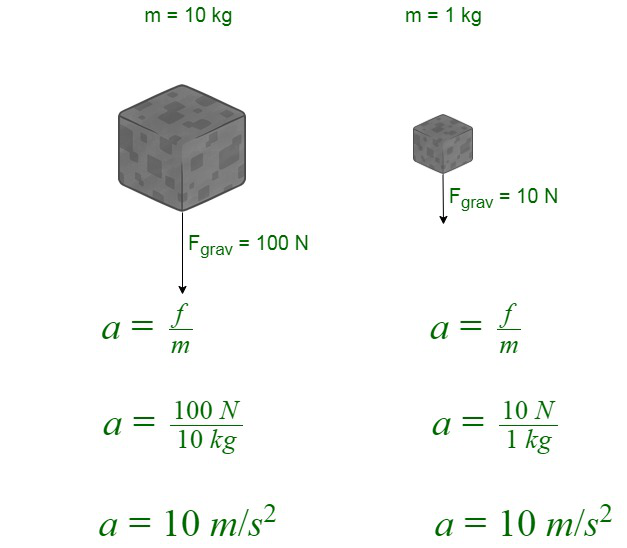### Factor affecting the value of Acceleration due to Gravity

There are various facts that affects the value of g, that are:

• Variation of g with Height: The value of g is inversely to the height above the earth surface therefore, it decreases with increasing height.
• Variation of g with Depth:The value of g is directly to the depth below the earth surface therefore, it increases with increasing depth but at the Centre of the earth it becomes equal to zero.
• Variation of g due to shape of the Earth: The value of g at the equator is less than the value of g at the pole.
• Variation of g due to Rotation of Earth: The value of g decreases with the increase in the rotation of the earth.

Lets discuss each case in more detail as:

### Variation of g with Height

Consider a sample mass (m) at a height (h) above the earth’s surface. Now, the gravitational force exerted on the test mass is:

F = GMm / (R+h)2

where R and M are the radius and the mass of the earth. Then, the acceleration due to gravity at a certain height is ‘h’. So,

mgh = GMm / (R+h)2

⇒ gh = GM / [R2 (1+ h/R)2]                                                                                        ……(1)

Now, the value of g is,

g = GM/R2                                                                                                                  ……(2)

On dividing equation (2) and (1) we get,

gh = g (1+h/R)-2                                                                                                        ……(3)

This is the acceleration due to gravity at a height above the earth’s surface. According to the above formula, the value of g decreases with increasing height of an object and becomes zero at infinite distance from the earth.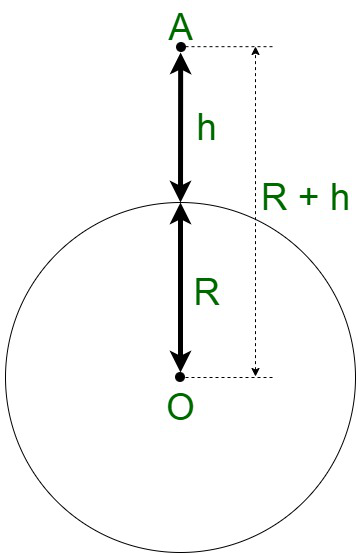### Variation of g with Depth

Suppose we consider a body of mass m is at a point B where B is at a depth of h from the earth’s surface, and its distance from the center is R – h.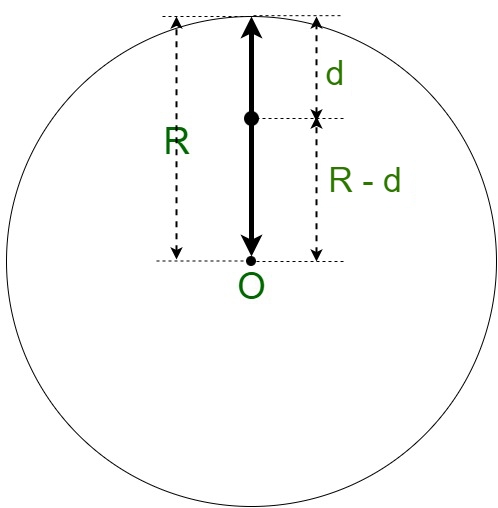Now, we have,

gd = g (R – d)/R

The acceleration due to gravity (gd), at this depth, is given by,

h < R

or

(1 – h/R) < 1

Therefore, gd < g

And this acceleration, (gd), decreases as we move towards the center of the earth which is experienced only when we move very deep towards the center of the earth. Hence, the value of g changes with height and depth.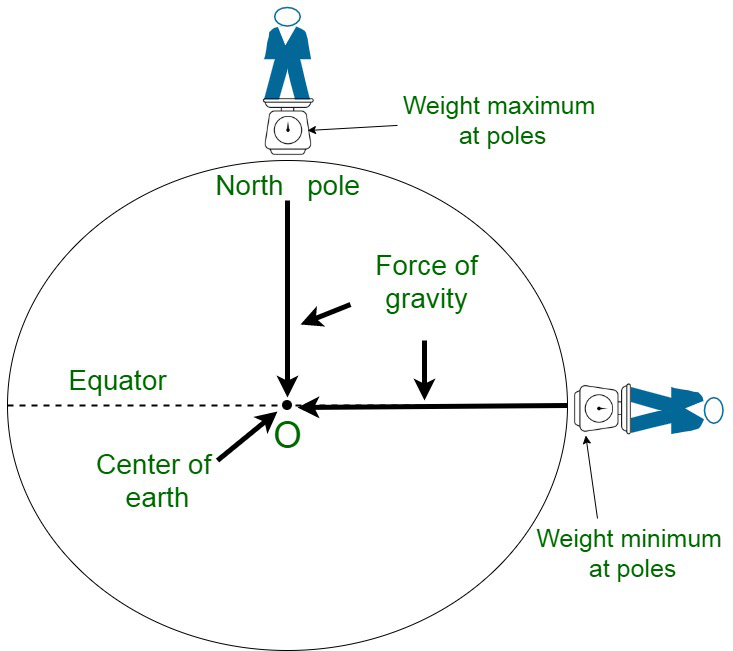But the value of g changes even on the surface of the earth also. For example, g is the highest on the poles and lowest on the equator.

### Variation of g due to Shape of the Earth

The earth is not perfectly spherical but is an oblate spheroid. The polar radius (radius near poles) earth’s is 21 km smaller than its equatorial radius (near the equator). Earth is not spherical, but actually, it is bulged out as shown below.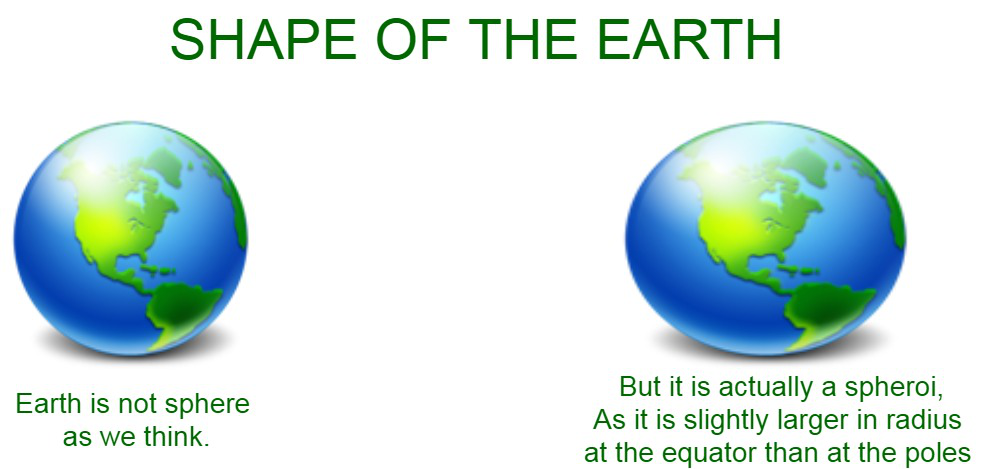As per the formula derived, the acceleration due to gravity is inversely proportional to the square of the radius of the earth. The radius of the earth at the equator is more; at the equator, g is less. This is the reverse in the case of poles.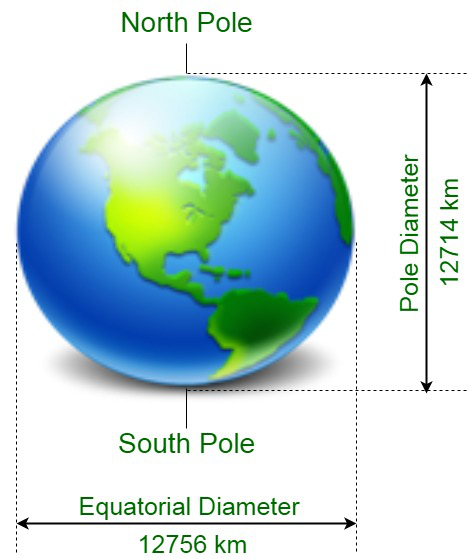Suppose we consider the shape of the earth as slightly elliptical. So, we will have different distances from the pole and the equator from the center.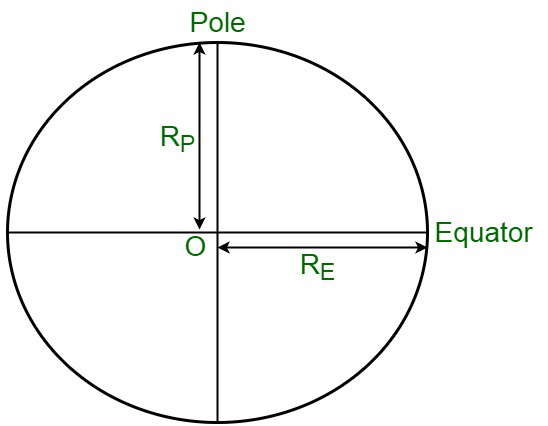So, the distance between the pole (RP) and the equator (RE) from the center is as,

RE > RP

And, from observation, we have the relation between RE and RP as,

g ∝ 1/R2

Then, if we consider G and M as constants in the acceleration formula, then,

gP ∝ 1/RP2 and

gE ∝ 1/RE2

So, the gravitational accelerations on the equator and pole are given by,

gP > gE

Here, from the distances between the poles and the equator, we have the relationship of gravitational acceleration as,

gP / gE = RE2 / RP2

Hence, the gravitational acceleration at the equator is less than the gravitational acceleration at the pole.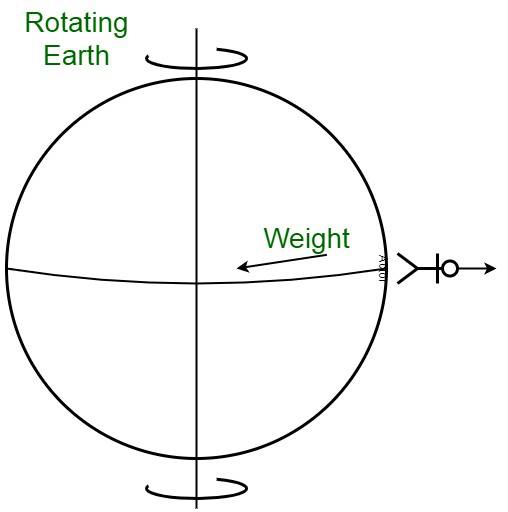### Variation of g due to Rotation of Earth

The variation in g due to the centrifugal force acting on the rotation of the earth. When the earth is rotating, all the objects tend to experience a centrifugal force that won’t act in the direction of gravity.

Consider a sample mass (m) that is located on a latitude that forms an angle with the equator. As we have seen, when a body rotates, every particle in the body moves in a circular motion around the axis of revolution. In this instance, the earth rotates with a constant angular velocity, while the test mass travels with an angular velocity in a circular path of radius ‘r’.

Because this is a non-inertial frame of reference, there is a centrifugal force acting on the sample mass (mr2). Gravity pulls the test mass towards the center of the planet (mg). Because both of these forces act from the same point, they are referred to as co-initial forces, and because they fall on the same plane, they are referred to as co-planar forces.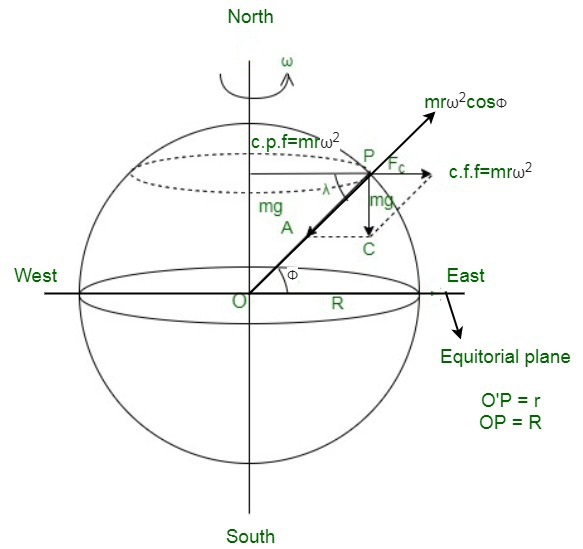According to the parallelogram rule of vectors, if two coplanar vectors form two sides of a parallelogram, the resultant of those two vectors will always be along the parallelogram’s diagonal. Using the parallelogram law of vectors, we can calculate the magnitude of the apparent value of gravitational force at the latitude:(mg′)2 = (mg)2 + (mrω2)2 + 2(mg) (mrω2) cos(180 – θ)

where r is the radius of the circular path followed which is, r = R cosθ.

Therefore, the above expression becomes,

g’ = g – Rω2 cos2θ

where g′ is the apparent amount of acceleration due to gravity at the latitude owing to earth rotation, and g is the real value of gravity at the latitude without considering earth rotation.

Also, both g and g’ are related at different position on earth given as,

### Sample Problem

Problem 1: A man, Ravi, weighs on the surface of the earth. At what height will Ravi’s weight become half, which is reduced to W/2? Assume the radius of the earth to be R.

Solution:

Given that,

Wh = W / 2

Radius of the Earth = R

We have to find R,Thus, r = R + h

or,

h = r – R

= 1.4.14R – R

= 0.414R

Therefore, at the height of 0.414R, a man’s weight becomes half his weight on the surface of the earth.

Problem 2: The earth is assumed to be spherically homogeneous for all practical purposes. Find the acceleration due to gravity at a depth of 2000 km beneath the surface of the earth. [Use: R = 6400 km and g = 9.8 m/s2.]

Solution:

Given that:

The depth, d = 2000 km,

The radius of the earth, R = 6400 km.

We have to find acceleration due to gravity gdTherefore, the acceleration at a depth of 2000 km below the surface of the earth is 6,738 m/s2.

Problem 3: Point P is located at the surface of the earth, with a radius of 6400 km. The particle accelerates downward with a constant acceleration to reach a point Q situated at a depth d is equal to 1600 km from the earth’s surface. Find the reduction witnessed in the acceleration due to gravity at Q.

Solution:

Given that,

The depth, d = 1600 km,

The radius of the earth, R = 6400 km,

Also the acceleration due to gravity, g = 9.8 m/s2

We have to find the decrease in value of acceleration due to gravity i.e. g – gdHence,

g – gd = 9.8 m/s2 – 7.35 m/s2

= 2.45 m/s2

Therefore, the decrease in acceleration due to gravity is 2.45 m/s2.

Problem 4: A person weighs 50 kg on Earth. On the surface of Neptune, where the acceleration of gravity is approximately 1.2 times as great as that on Earth, what will be the person’s mass?

Solution:

The person will weigh 50 kg on the surface of Neptune because the force of gravity changes the weight of the object and not it’s mass. The mass of an object stays constant regardless of the forces acting upon it.

Problem 5: It has been noted that at a place above the earth’s surface, the value of g is 0.2 m/s2. Determine this height above the earth’s surface at which the given g is obtained.

Solution:

Consider the height above earth surface be h’.

Therefore,

g′ = g (1− 2h / R)

or ​

g′ / g = (1− 2h / R)

Substitute the given values in the above expression as,

0.2 m/s2 / 9.8 m/s2 = (1− 2h / R)

2h / R = 48 / 49

h = 3134. 7 km

= 3134.7 km

Attention reader! All those who say programming isn’t for kids, just haven’t met the right mentors yet. Join the  Demo Class for First Step to Coding Course, specifically designed for students of class 8 to 12.

The students will get to learn more about the world of programming in these free classes which will definitely help them in making a wise career choice in the future.

My Personal Notes arrow_drop_up